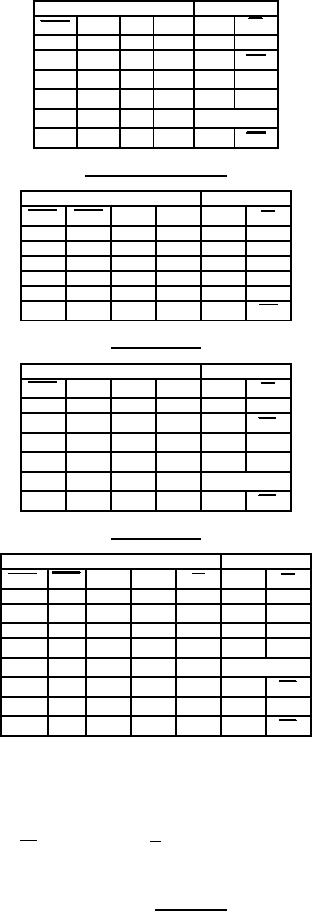Click here to make tpub.com your Home Page Page Title: Figure 2. Truth tables. Back | Up | NextWeb www.tpub.comHome

Information Categories
Aerographer
Automotive
Aviation
Construction
Diving
Draftsman
Engineering
Electronics
Food and Cooking
Logistics
Math
Medical
Music
Nuclear Fundamentals
Photography
ReligionMIL-M-38510/653B
Device type 01
Inputs
Outputs
CLR
CLK
J
K
Q
Q
L
X
X
X
L
H
H
L
L
Q0
Q0
H
H
L
H
L
H
L
H
L
H
H
H
H
Toggle
H
H
X
X
Q0
Q0
Device types 02 and 52
Inputs
Outputs
PRE
CLR  CLK
D
Q
Q
L
H
X
X
H
L
H
L
X
X
L
H
L
L
X
X
H*
H*
H
H
H
H
L
H
H
L
L
H
H
H
X
X
Q0
Q0
Device type 03
Inputs
Outputs
CLR
CLK
J
K
Q
Q
L
X
X
X
L
H
H
L
L
Q0
Q0
H
H
L
H
L
H
L
H
L
H
H
H
H
Toggle
H
H
X
X
Q0
Q0
Device type 04
Inputs
Outputs
PRE
CLR  CLK
J
K
Q
Q
L
H
X
X
X
H
L
H
L
X
X
X
L
H
L
L
X
X
X
H*
H*
L
L
L
H
H
H
H
L
Toggle
H
H
H
H
L
H
Q0
Q0
H
H
H
L
H
H
H
H
L
X
X
Q0
Q0
* = This configuration is nonstable
X = Pins have no effect on output
H = High level voltage
L = Low level voltage
= Low-to-high transition of the clock
= High-to-low transition of the clock
Q0 (Q0) = The level of Q (Q) before the indicated steady-state
input conditions were established
FIGURE 2. Truth tables.
15